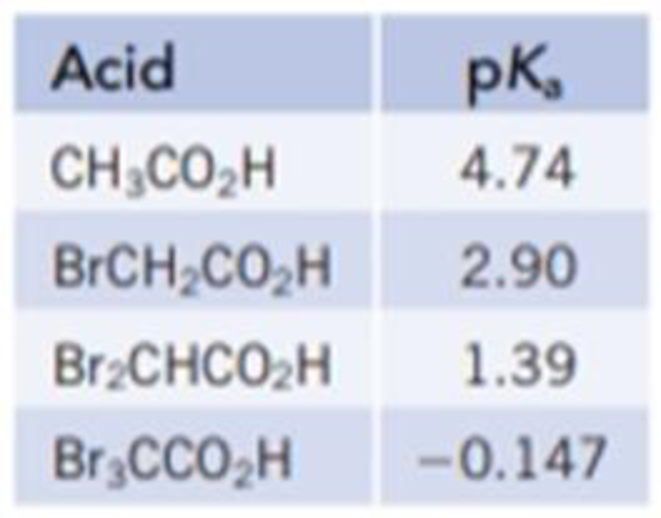Chapter 16, Problem 112IL

Chapter
Section
Textbook Problem

The data below compare the strength of acetic acid with a related series of acids, where the H atoms of the CH3 group in acetic acid are successively replaced by Br.(a) What trend in acid strength do you observe as H is successively replaced by Br? Can you suggest a reason for this trend? (b) Suppose each of the acids above was present as a 0.10 M aqueous solution. Which would have the highest pH? The lowest pH?

(a)

Interpretation Introduction

Interpretation:

The trend in acid strength has to be explained.

Concept Introduction:

A carboxylic acid can behave as a stronger bronsted acids when the hydrogen atom of alpha carbon is replaced by an anion. This condition is enhanced by:

(1) The presence of electronegative atom.

(2) The Greater positive formal charge on the central atom.

(3) The Possibility of resonating structures for the anion that delocalize the negative charge on the anion, which lead to stability.

Explanation

The acid strength increases as the number of electronegative atoms (bromine) increase on methyl group by successive replacement of hydrogen atoms. An electronegative substituent stabilizes the negative charge on anion. The acidity of Acetic acid is enhanced, if electronegative atom, Br replaces the hydrogen atoms in the methyl (CH3) group. Therefore, the Br3CCOO anion is more stable than the CH3COO anion

(b)

Interpretation Introduction

Interpretation:

The trend in acid strength and the acid which have the highest pH and which have the lowest pH is to be stated.

Concept Introduction:

The pH is a measure of Hydronium ion concentration. The expression for pH is,

pH=log[H3O+] (1)

Higher the value of Ka, stronger the acid and for the acidic solution pH<7, which means higher the value of Ka, smaller the value of pH.

Still sussing out bartleby?

Check out a sample textbook solution.

See a sample solution

The Solution to Your Study Problems

Bartleby provides explanations to thousands of textbook problems written by our experts, many with advanced degrees!

Get Started